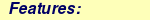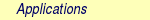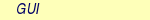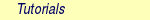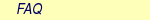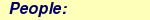MOLCAS manual:Next: 5.3 Computing excited states Up: 5. Problem Based Tutorials Previous: 5.1 Electronic Energy at

# 5.2 Optimizing geometries

It is now useful to explore potential energy surfaces (PES) and optimize the molecular geometry for specific points along the PES. Different cases are discussed including a way to obtain the optimal geometry in a minimum energy search, to obtain a transition-state structure connecting different regions of the PES, to find the crossing between two PES where the energy becomes degenerate, or to map the minimum steepest-descent energy path (MEP) from an initial point to the final a minimum energy geometry as the PES progresses in a downward manner.

All these types of searches can be performed either by fully optimizing all degrees of freedom of the system or by introducing certain restrictions. MOLCAS 8.2 can perform geometry optimizations at the SCF (RHF and UHF), DFT (RHF and UHF based), CASSCF (CASSCF and RASSCF) levels of theory, where efficient analytical gradients are available and at the CASPT2 and other correlated levels where numerical gradients are used.

Geometry optimizations require many cycles, in which the electronic energy is estimated at a specific level of calculation followed by calculation of the gradient of the energy with respect to the geometric degrees of freedom (DOF). With this information at hand, the program must decide if the molecule is already at the final required geometry (i.e. gradient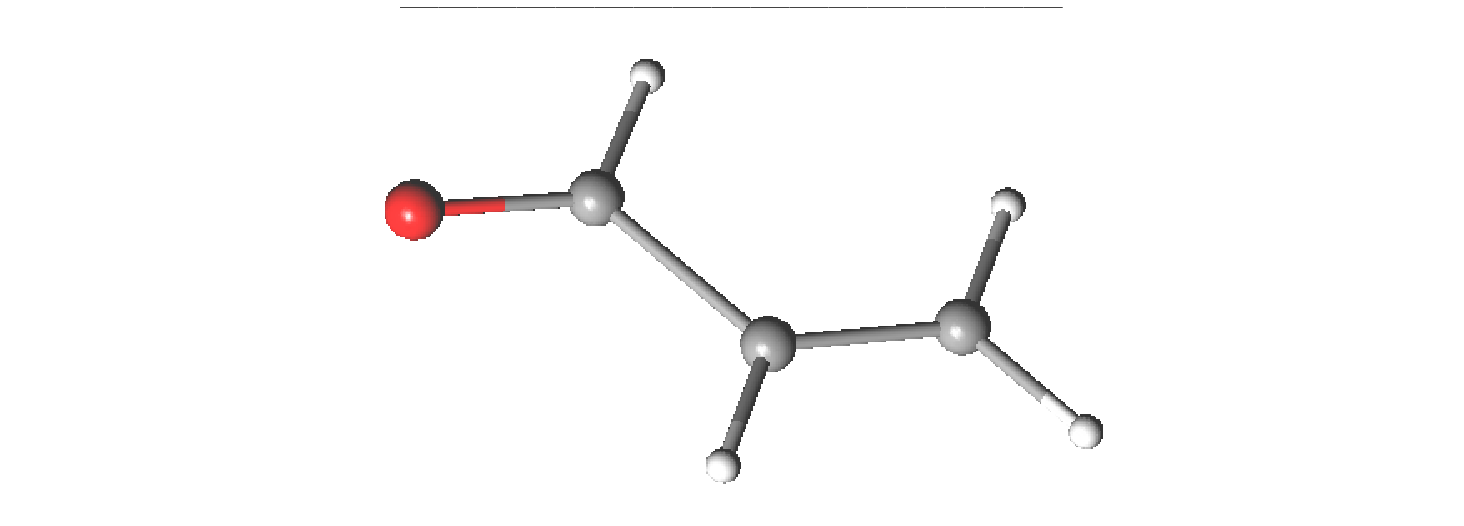0 for all DOF) indicating a minimum in the PES or if the geometry must be modified and continue the cycle. The input file should, therefore, be built in a way that allows a loop over the different programs.

The general input commands Do while and Enddo control the loop and program input is inserted within these commands. Instructions for the number of maximum iterations allowed and the type of output required can also be added. (see section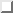)

All examples previously discussed, use COORD keyword, but it also possible to use native format, where symmetry unique atoms are specified (SYMMETRY) and provide generators to construct all atoms in the molecule.

The selected example describes geometry optimization of the water molecule at the SCF RHF level of calculation:

3
coordinates  for  water  molecule  NOT  in  equilibrium
O  0.000000  0.000000  0.000000
H  0.758602  0.000000  0.504284
H  0.758602  0.000000  -0.504284

*SCF  minimum  energy  optimization  for  H2O
*File:  SCF.minimum_optimization.H2O
*
&GATEWAY
Title=  H2O  minimum  optimization
coord=Water_distorted.xyz
basis=ANO-S-MB
group=C1

>>>  Do  while
&SEWARD  ;&SCF;  &SLAPAF
>>>  EndDo

The sequence of programs employed includes GATEWAY which is external to the loop, followed by SEWARD, SCF, and SLAPAF. SEWARD computes the integrals, SCF program computes the RHF energy and wave function, and SLAPAF will control the calculation of gradients and estimate if the calculation has already finished or needs to proceed to a new nuclear geometry for the next iteration. Automatically, a file named \$Project.geo.molden will be generated in \$WorkDir containing all the geometric steps contained in the optimization process. MOLDEN or LUSCUS can then read this file to display the individual molecular geometries which form the optimization cycle.

Using another reference wave function can be simply performed by changing the sequence of programs. For instance, we can perform an UHF calculation of the H2O+ cation:

*UHF  minimum  energy  optimization  for  H2O+
*File:  UHF.minimum_optimization.H2Oplus
*
&GATEWAY
Title=  H2O  minimum  optimization
coord=Water_distorted.xyz
basis=ANO-S-MB
group=C1
>>  Do  while

&SEWARD
&SCF;  Title="H2O  minimum  optimization";  UHF;  Charge=1
&SLAPAF

>>  EndDo

The same procedure can be followed if we pretend to perform a DFT geometry optimization:

*DFT  minimum  energy  optimization  for  H2O
*File:  DFT.minimum_optimization.H2O
*
&GATEWAY
Title=  H2O  minimum  optimization
coord=Water_distorted.xyz
basis=ANO-S-MB
group=C1

>>>  Export  MOLCAS_MAXITER=100
>>>  Do  while

&SEWARD
&SCF  ;  Title="H2O  minimum  optimization";  KSDFT=B3LYP
&SLAPAF  &END

>>>  EndDo

Once an energy minimum is found based on the calculation of gradients, it is necessary to ensure that the geometry really is a minimum energy point. This can be only accomplished by computing second derivatives of the energy (i.e. the Hessian). MOLCAS can compute analytical Hessians for SCF and single state CASSCF wave functions. For other methods, numerical procedures can be used to compute the Hessian. Once the Hessian is computed, vibrational frequencies are calculated, and Statistical Mechanics is used to obtain thermodynamic properties. At a true energy minimum, there will be 3N-6 real frequencies Program MCKINLEY computes second derivatives of a predefined (SCF or CASSCF) wave function, while MCLR performs the vibrational and statistical analyses. MOLCAS simply requires input for the MCKINLEY program to perform the entire calculation by using keywords Perturbation and Hessian, while program MCLR will be called automatically but requires no input. The full set of calculationsi is included below first a geometry optimization followed by the calculation of a Hessian.

*SCF  minimum  energy  optimization  plus  hessian  of  the  water  molecule
*File:  SCF.minimization_plus_hessian.H2O
*
&GATEWAY
Title=  H2O  minimum  optimization
coord=Water_distorted.xyz
basis=ANO-S-MB
group=C1

>>>  Export  MOLCAS_MAXITER=100
>>>  Do  while

&SEWARD
&SCF;  Title="H2O  minimum  optimization"
&SLAPAF

>>>  EndDo

&MCKINLEY

Note that MCKINLEY input above is placed after EndDo, and, therefore, is external to the looping scheme. Once the geometry optimization at the desired level of theory has finished, the Hessian will be computed at the final geometry. In general, any calculation performed using a \$WorkDir directory where a previous geometry optimization has taken place will use the last geomtry calculated from that optimization as the input geometry even if SEWARD input is present. To avoid that, the only solution is to remove the communication file RUNFILE where the geometry is stored. Note also, that the frequencies are computed in a cartesian basis, and that three translational and three rotational frequencies which should be very close to zero are included in the output file. This is not the case when numerical gradients and Hessians are used. In particular, for water at its minimum energy structure three (3N-6) real vibrational frequencies. By default, in \$WorkDir a file \$Project.freq.molden is generated containing the vibrational frequencies and modes, which can be visualized by MOLDEN.

A new level of theory, CASSCF, is introduced here which is especially suited for geometry optimizations of excited states discussed in the next chapter. A geometry optimization is performed to illustrate a broader range of possibilities including the imposition of a geometric restrain that the HOH angle in water should be constrained to 120o during the optimization. This means that only the O-H bond distances be optimized in this partial minimization. The restriction is indicated in in GATEWAY by invoking the keyword Constraints and ending with the keyword End of Constraints. The names of variables corresponding to geometrical variables in either internal or Cartesian coordinates that are to be constrained are placed between these two keywords. (see nomenclature in section) In the case of H2O, the H1-O-H2 angle is fixed at 120o, so a variable, a, is first defined with the keywork (Angle), which relates it to the H1-O1-H2 angle, followed by the second keyword, Value, where the variable a is specified as 120o. It is not required that the initial geometry is 120o, only that the final result for the calculation will become 120o.

Note that the RASSCF program requires initial trial orbitals, and those which are automatically generated by SEWARD are used. The resulting CASSCF wave function includes all valence orbitals and electrons.

*CASSCF  minimum  energy  optimization  of  the  water  molecule  with  geometrical  restrictions
*File:  CASSCF.minimum_optimization_restricted.H2O
&GATEWAY
Title=  H2O  minimum  optimization
coord=Water_distorted.xyz
basis=ANO-S-MB
group=C1
Constraint
a  =  Angle  H2  O1  H3
Value
a  =  90.  degree
End  of  Constraints

>>>  Do  while

&SEWARD
&RASSCF;  nActEl=8  0  0;  Inactive=1;  Ras2=6
&SLAPAF

>>>  EndDo

Other more flexible ways to impose geometric restrictions involve the specification of which internal coordinates should remain fixed and which should change. In the next example, the bond lengths are forced to remain fixed at their initial distance (here 0.91 Å), while the bond angle, having an initial of 81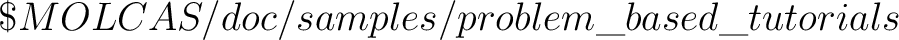, is optimized.

*DFT  minimum  energy  optimization  of  the  angle  in  the  water  molecule  at  fixed  bond  lengths
*File:  DFT.minimum_optimization_restricted.H2O
*
&GATEWAY
Title=  H2O  minimum  optimization
coord=Water_distorted.xyz
basis=ANO-S-MB
group=C1

>>>  EXPORT  MOLCAS_MAXITER=100
>>>  Do  while

&SEWARD;  &SCF;  Title="H2O  restricted  minimum";  KSDFT=B3LYP
&SLAPAF
Internal  Coordinates
b1  =  Bond  O1  H2
b2  =  Bond  O1  H3
a1  =  Angle  H2  O1  H3
Vary
a1
Fix
b1
b2
End  of  Internal

>>>  EndDo

In the final output, the two O-H bond lengths remain at the initia values, while the H1-O1=H2 angle is optimized to a final angle of 112o.

The next step entails the computation of a transition state, a structure connecting different regions of the potential energy hypersurface, and is a maximum for only one degree of freedom. The most common saddle points have order one, that is, they are maxima for one of one displacement and minima for the others. The simplest way to search for a transition state in MOLCAS is to add the keyword TS to the SLAPAF input. Keyword PRFC is suggested in order to verify the nature of the transition structure. Searching for transition states is, however, not an easy task. An illustration of the input required for transition state optimization for water at the DFT level is given below:

3
water  in  Transition  state  in  bohr
O1  0.750000  0.000000  0.000000
H2  1.350000  0.000000  1.550000
H3  1.350000  0.000000  -1.550000

*DFT  transition  state  optimization  of  the  water  molecule
*File:  DFT.transition_state.H2O
*
&Gateway
Coord=Water_TS.xyz
Basis=ANO-S-VDZ
Group=C1
>>>  Do  while

&SEWARD
&SCF;  Title="H2O  TS  optimization";  KSDFT=B3LYP
&SLAPAF  ;  ITER=20  ;  TS

>>>  EndDo

The initial coordinates were chosen in units of Bohr, to illustrare that this is the default case. The optimal geometry for ground state of water is a structure with C2v symmetry. A transition state has been found with a linear HOH angle of 180o. In many cases, there may be a clue along the energy pathway for a chemical reaction about the nature of the transition state structure, which typically represents an intermediate conformation between reactants and products. If this turns out to be the case, it is possible to help the optimization process proceed toward an informed guess, by invoking the keyword FindTS in SLAPAF. FindTS must to be accompanied with a definition of constrained geometric definitions. SLAPAF will guide the optimization of the transition state towards a region in which the restriction is fulfilled. Once there, the restriction will be released and a free search of the transition state will be performed. This technique is frequently quite effective and makes it possible to find difficult transition states or reduce the number of required iterations. Here, an example is provided, in which the initial geometry of water is clearly bent, and a trial restraint is imposed such that the angle for the transition state should be near 180o. The final transition state will, however, be obtained without any type of geometrical restriction.

*DFT  transition  state  optimization  of  the  water  molecule  with  geometrical  restrictions
*File:  DFT.transition_state_restricted.H2O
*
&Gateway
Coord=Water_TS.xyz
Basis=ANO-S-VDZ
Group=C1
Constraints
a  =  Angle  H2  O1  H3
Value
a  =  180.0  degree
End  of  Constraints

>>>  Do  while

&SEWARD
&SCF;  Title="H2O  TS  optimization";  KSDFT=B3LYP
&SLAPAF  ;FindTS

>>>  EndDo

The CASPT2 geometry optimizations are somewhat different because ALASKA is not suited to compute CASPT2 analytical gradients. Therefore the ALASKA program is automatically substituted by program NUMERICAL_GRADIENT, which will take care of performing numerical gradients. From the user point of view the only requirement is to place the CASPT2 input after the RASSCF input. The CASSCF wave function has of course to be generated in each step before performing CASPT2. To compute a numerical gradient can be quite time consuming, although it is a task that can be nicely parallelized. In a double-sided gradient algorithm like here a total of 6N-12+1 CASPT2 calculations are performed each pass of the optimization, where N is the number of atoms.

*CASPT2  minimum  energy  optimization  for  water
*File:  CASPT2.minimum_optimization.H2O
*
&GATEWAY
coord=Water_distorted.xyz
basis=ANO-S-MB
group=C1

>>>  Do  while

&SEWARD
&RASSCF;  Title="H2O  restricted  minimum";  nActEl=8  0  0;  Inactive=1;  Ras2=6
&CASPT2;  Frozen=1
&SLAPAF

>>>  EndDo

The use of spatial symmetry makes the calculations more efficient, although they may again complicate the preparation of input files. We can repeat the previous CASPT2 optimization by restricting the molecule to work in the C2v point group, which, by the way, is the proper symmetry for water in the ground state. The GATEWAY program (as no symmetry has been specified) will identify and work with the highest available point group, C2v. Here the molecule is placed with YZ as the molecular plane. By adding keyword Symmetry containing as elements of symmetry the YZ (symbol X) and YX (symbol Z), the point group is totally defined and the molecule properly generated. From that point the calculations will be restricted to use symmetry restrictions. For instance, the molecular orbitals will be classified in the four elements of symmetry of the group, a1, b1, b2, and a2, and most of the programs will require to define the selection of the orbitals in the proper order. The order of the symmetry labels is determined by SEWARD and must be checked before proceeding, because from that point the elements of symmetry will be known by their order in SEWARD: a1, b1, b2, and a2, for instance, will be symmetries 1, 2, 3, and 4, respectively. SCF does not require to specify the class of orbitals and it can be used as a learning tool.

*CASPT2  minimum  energy  optimization  for  water  in  C2v
*File:  CASPT2.minimum_optimization_C2v.H2O
*
&GATEWAY
Title=  H2O  caspt2  minimum  optimization
Symmetry=  X  Z
Basis  set
O.ANO-S...2s1p.
O  0.000000  0.000000  0.000000  Angstrom
End  of  basis
Basis  set
H.ANO-S...1s.
H1  0.000000  0.758602  0.504284  Angstrom
End  of  basis

>>>  EXPORT  MOLCAS_MAXITER=100
>>>  Do  while

&SEWARD
&RASSCF;  nActEl=8  0  0;  Inactive=1  0  0  0;  Ras2=3  1  2  0
&CASPT2;  Frozen=1  0  0  0
&SLAPAF  &END

>>>  EndDo

Thanks to symmetry restrictions the number of iterations within NUMERICAL_GRADIENT has been reduced to five instead of seven, because many of the deformations are redundant within the C2v symmetry. Also, symmetry considerations are important when defining geometrical restrictions (see sectionsand). (see online manual).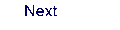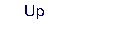Next: 5.3 Computing excited states Up: 5. Problem Based Tutorials Previous: 5.1 Electronic Energy at